# How the Popular ML models workBy: Divya Bora

May 4, 2021

“Machine Learning is focused on computers learning and acting like a human, and improve learning with time autonomously by feeding data and information in the form of observations and real-world interactions.”

Machine Learning (ML) is considered an application of Artificial Intelligence (AI) that enables systems to automatically learn and improve without being explicitly programmed. An algorithm is a sequence of statistical processing steps. Machine learning consists of algorithms trained to find features or patterns from huge chunks of data to make predictions and decisions based on the new data. Better algorithms lead to more accurate predictions of the data processed.

To understand how ML works, let us discuss four basic steps performed while building an ML application.

Step 1: Select and prepare a training data set

Training data set is the data required by the ML model to solve the problem it was programmed for. This training data set can either be labeled (specifies the features and classifications the model needs to identify) or unlabeled (the model will extract the features itself and assign them classifications). Training data should be randomized, checked for biases or imbalances, and de-duped. Further, the training data set is divided into two subsets: a training subset (used to train the application) and an evaluation/testing subset (used to test and refine the application).

Step 2: Choose an algorithm appropriate for the selected training data set

As defined earlier, an algorithm is a set of statistical processing steps. However, the type of algorithm depends on the type and amount of data provided by the training data set and the nature of the problem being solved by the application. Common types of ML algorithms used with labeled data are Regression algorithms like logistic regression, linear regression and support vector machines, decision trees, and instance-based algorithms like K-Nearest Neighbor. Common types of machine learning algorithms used with unlabeled data are clustering algorithms like K-means, TwoStep and Kohonen clustering, Association algorithms, and Neural networks.

Step 3: Training the algorithm to create the model

While training is not a one-time process, it involves the constant running of variables through the algorithm, comparing the output with the expected results, altering weights and biases within the algorithm to yield a more accurate result, and running the variables again algorithm returns the expected result frequently. The resulting accurately trained algorithm is the required machine learning model.

Step 4: Using and improving the model

This is the final step where we use the trained model with new data, and in the best-case scenario, the model will display improvement in accuracy and effectiveness over time. The origin of the new data varies as it relies on the problem being solved by the model.

### MACHINE LEARNING MODELS

Each type of ML algorithm consists of machine learning models. Machine learning algorithms can be categorized as: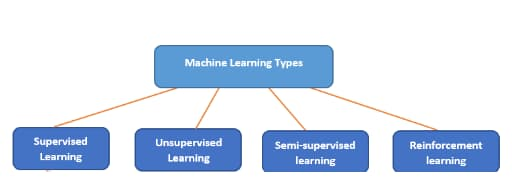1. Supervised Machine Learning Algorithms

This algorithm consists of dependent variables (or target variables) that must be predicted based on given independent variables (or predictors). A function is created to map inputs to the desired outputs, and this training process continues until the model attains the desired accuracy. Some popular supervised learning models are:

1.1 K-Nearest Neighbor (k-NN) Model

It is one of the most popular classification models. It compares Euclidean distance between the provided data set and new observation where “k” is the number of neighbors it corresponds to the distance to. The closeness of the new observation to most particular class neighbors determines which class the point will fall into, and usually, k is an odd number to prevent ties.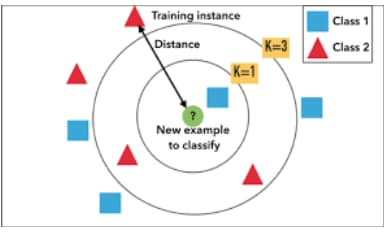1.2 Random Forest Model

This type of model is an ensemble method that operates by creating various decision trees and generates classifications for individual trees. A forest is a collection of decisions. To further classify a new object based on its attributes, each tree gives a classification and votes for that class. As a result, the forest then chooses the classification having the most votes.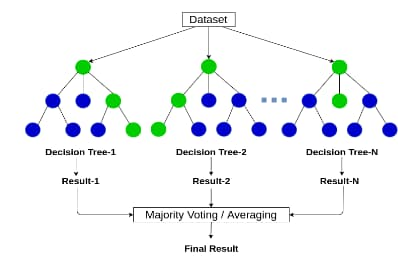1.3 Decision Tree

A decision tree is like a flowchart-type model consisting of conditional control statements comprising decisions and probable consequences. The output relates to the labeling of unforeseen data. In the decision tree, leaf nodes correspond to class labels, and internal nodes represent the attributes; these decision trees are used to resolve problems involving discrete attributes and boolean functions.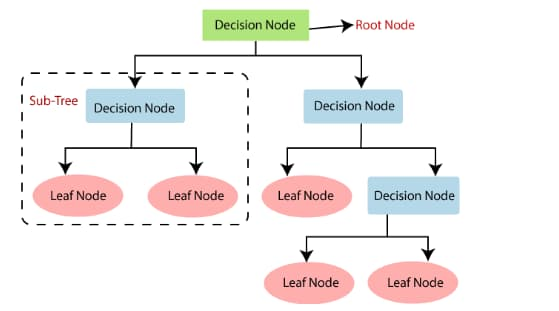1.4 Naive Bayesian Model

This model is based on the Bayes’ theorem. It assumes that the presence of a particular feature in a specific class is completely unrelated to the presence of another feature. It is generally used for large finite datasets and assigns class labels using a direct acyclic graph. This graph consists of one parent node and multiple children nodes where each child is independent and separate from their parent.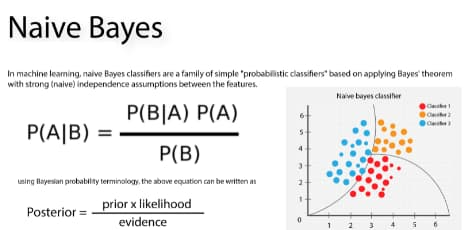2. Unsupervised Machine Learning Algorithms

This algorithm does not consist of any dependent variables to predict the outputs as it uses an unlabeled dataset. Some popular unsupervised learning models are:

2.1 K-Means Model

This is an interactive clustering algorithm that assists in finding the highest value for every iteration. First, k (the desired number of clusters) is selected. Then, data points need to be clustered into k groups. A higher value of k means small groups with high granularity, and a lower k means larger groups with lower granularity. The output is a group of labels and assigns data points to one of the k groups. Groups are defined by creating a centroid per group, and these centroids act as the heart of the cluster, capturing the points nearest to them and adding them to the cluster. K-means clustering is further divided into two subgroups:

a) Agglomerative Clustering

This type of K-means clustering begins with a fixed number of clusters and allocates the given data into a specific number of clusters. K (the number of clusters) is not required as an input in this model. Here, each given data forms a single cluster and uses some distance measures to reduce the number of clusters in each iteration by merging processes. Finally, one big cluster is left, which contains all the objects.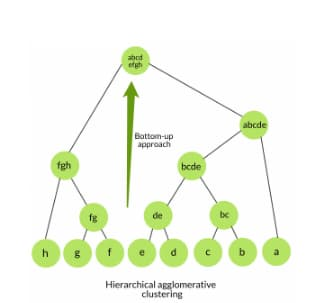b) Dendrogram

This type of K-means clustering method represents a possible cluster. Here, the height of the dendrogram will indicate the similarity between two join clusters. When it is closer to the end of the process, more similar clusters find the group from the dendrogram unnaturally and mostly subjective.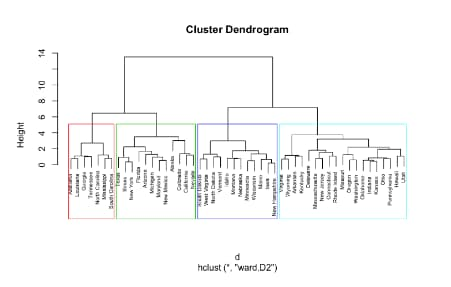2.2 Hierarchical Clustering

This is a type of algorithm that creates a hierarchy of clusters. In this type of clustering, all the data is assigned to a cluster of its own, where two close clusters are part of the same cluster. Finally, the algorithm ends when only a single cluster is left.

2.3 Principal Components Analysis

This is the model used when one requires a higher-dimensional space. One needs to select the basis for space and specify the 200 most important scores for that basis. This base is known as the principal component. The selected subset constitutes a new space generally smaller in size than the original space, and data complexity is maintained.3. Semi-Supervised Machine Learning Algorithms

This algorithm lies between the supervised and unsupervised learning algorithms as it makes use of both labeled and unlabeled datasets for training and improves its accuracy.

4. Reinforcement Machine Learning Algorithms

This algorithm trains the machine to make appropriate decisions by exposing the machine to a training environment where the machine learns by the trial and error method and improves its decision-making capabilities. Some popular reinforcement learning models are:

4.1 Markov Decision Process

This is a model that predicts outcomes based on the given information provided by the current state (a set of tokens representing the agent’s state) and incorporates the characteristics of actions and motivations. At each step of the process, the decision-maker may choose to take an available action in the current state, which results in the progress of the model, offering the decision-maker a reward in return. Using this model optimizes the actions taken within an environment to maximize the potential reward and determine the optimal balance between exploration and exploitation. Generally, the Markov Decision Process is used when the probabilities and rewards of an outcome are unspecified or unknown.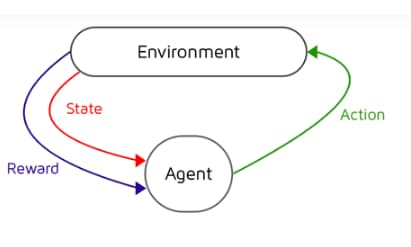4.2 Q Learning

This is a model-free algorithm that learns the value of an action in a particular state and handles issues with stochastic transitions and rewards without requiring adaptations. Q-values (also known as action values) are iteratively used to improve the behavior of the learning agent. Q is the function that the algorithm computes and the expected rewards for an action taken in a particular state. It searches for an optimal policy to maximize the expected value of the total reward over all the successive steps beginning from its current state. It can identify an optimal action-selection policy for any given infinite exploration time and a partly random policy.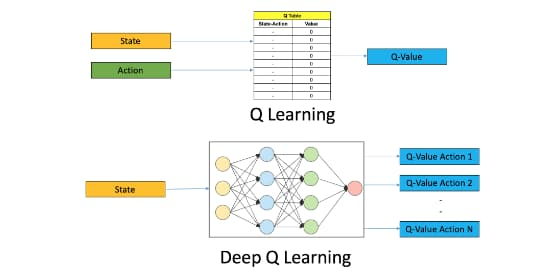To get a deeper understanding of Machine Learning, one can proceed with Data Science and Analytics, and to gain hands-on training in the domain, one can move forward with Azure Machine Learning.

REFERENCES